Courses

# Test: Two And Three Force Members

## 15 Questions MCQ Test Engineering Mechanics | Test: Two And Three Force Members

Description
This mock test of Test: Two And Three Force Members for Mechanical Engineering helps you for every Mechanical Engineering entrance exam. This contains 15 Multiple Choice Questions for Mechanical Engineering Test: Two And Three Force Members (mcq) to study with solutions a complete question bank. The solved questions answers in this Test: Two And Three Force Members quiz give you a good mix of easy questions and tough questions. Mechanical Engineering students definitely take this Test: Two And Three Force Members exercise for a better result in the exam. You can find other Test: Two And Three Force Members extra questions, long questions & short questions for Mechanical Engineering on EduRev as well by searching above.
QUESTION: 1

### The difference between the two and the three force members is:

Solution:

The definition of the two force member only defines that the forces are being acted on the two points on the body. So does is the definition of the three forces members. The points of action of the three forces are three.

QUESTION: 2

### Determine the vertical components of the reaction on the beam at pin Q.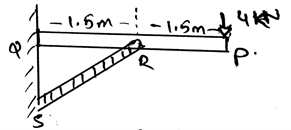Solution:

The summation of the forces needs to be zero. So does the summation of the moments need to zero. But talking about the angles, they not needed to zero. But the forces which are acting at particular angles, must needed to be equal to zero. The basic need of the forces to make the body in equilibrium.

QUESTION: 3

### Which of the following is correct?

Solution:

The force members doesn’t defines whether the moment will be applied or not. They are basically the forces only. Thus if there is a force applied on the body, there will be a moment produced by them. If not then probably the forces might be collinear.

QUESTION: 4

The three member forces are greatly influencing the moment than the two force members.

Solution:

The influence of the moment on the body is determined by the number of the forces acting on the body. It depend on the magnitude of the force. It also depend whether the forces are collinear or not. Thus the number of forces are not the only parameter for the determination of the moment.

QUESTION: 5

The three member forces apply three different moments and the two members apply two different moments on the body.

Solution:

Never judge the application of the moment of the force applied on the body by the number of the forces being acted on the body. What is two of the forces becomes collinear and are having a zero distance from the axis of rotation. Thus the three and two force member apply moments according to the situation, whether the distance is zero or not.

QUESTION: 6

For two force members to be in equilibrium when _____________

Solution:

Simply, for the equilibrium the net force must be zero. That is the forces must be collinear if equilibrium is to be established. And they must be in the opposite directions. They also must be of same magnitude, cancelling each other.

QUESTION: 7

Determine the force in the section RS.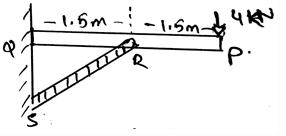Solution:

The summation of the forces needs to be zero. So does the summation of the moments need to zero. But talking about the angles, they not needed to zero. But the forces which are acting at particular angles, must needed to be equal to zero. The basic need of the forces to make the body in equilibrium.

QUESTION: 8

Moment equilibrium for the three force members will only be satisfied if_____________

Solution:

The moment equilibrium for the three force members is satisfies only when the three forces are concurrent. That is the forces form a parallel system. That is the net force on the three axis turns out to be zero. This will only happen if the forces are in the same plane.

QUESTION: 9

Which of the following statement for the three force system is true?

Solution:

The forces in the three force system is not always in the equilibrium. The equilibrium is established when the forces cancels out each other. Also when the net moment is zero. Then only the equilibrium is established in the three force system.

QUESTION: 10

Determine the horizontal components of the reaction on the beam at pin Q.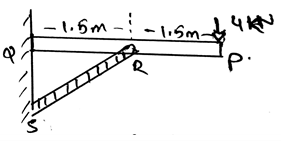Solution:

The summation of the forces needs to be zero. So does the summation of the moments need to zero. But talking about the angles, they not needed to zero. But the forces which are acting at particular angles, must needed to be equal to zero. The basic need of the forces to make the body in equilibrium.

QUESTION: 11

Determine the magnitude of the force F = 300j parallel to the direction of AB?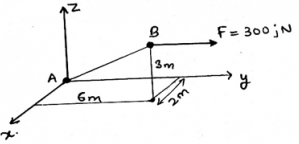Solution:

Force component in the direction parallel to the AB is given by unit vector 0.286i + 0.857j + 0.429k. Now (300j).(0.286i + 0.857j + 0.429k) = 257.1N. Just try to resolve the force into it’s particular components.

QUESTION: 12

All the forces need to be collinear in the three force system. Then only they will for the equilibrium state.

Solution:

The forces need to be collinear. If they are not so then the equilibrium is not possible. If they are parallel too, then it is assumed that they are meeting in the infinity. And hence the equilibrium is established between the forces. Thus the forces must be collinear.

QUESTION: 13

Determine the vertical components of the reaction on the beam at pin Q.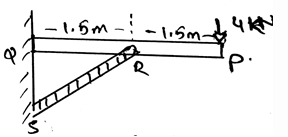Solution:

The summation of the forces needs to be zero. So does the summation of the moments need to zero. But talking about the angles, they not needed to zero. But the forces which are acting at particular angles, must needed to be equal to zero. The basic need of the forces to make the body in equilibrium.

QUESTION: 14

What is k.i?

Solution:

As the dot product of only the same Cartesian component is unity, i.e. i.i = 1 and j.j =1, rest all remaining dot product will give 0(i.j = 0 and j.k = 0). Cross product of the same plane vectors always give zero. And dot product of the same plane vector gives a scalar quantity.

QUESTION: 15

The three force system can also be in the equilibrium if_________

Solution:

Even if the forces components cancels each other than the body is said to be in equilibrium. Also it should satisfy whether the moments caused by the forces are equal to zero or not. This means if the moment caused and the net force is zero the body is in equilibrium.